MathScore EduFighter is one of the best math games on the Internet today. You can start playing for free!

## Circle Area - Sample Math Practice Problems

The math problems below can be generated by MathScore.com, a math practice program for schools and individual families. References to complexity and mode refer to the overall difficulty of the problems as they appear in the main program. In the main program, all problems are automatically graded and the difficulty adapts dynamically based on performance. Answers to these sample questions appear at the bottom of the page. This page does not grade your responses.

See some of our other supported math practice problems.

### Complexity=4, Mode=exact

Find the area in terms of π. Figures are not drawn to scale.
Type "pi" in for π. Example: "7π m2" as "7pi sq m".

 1.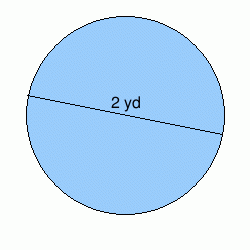Area: 2.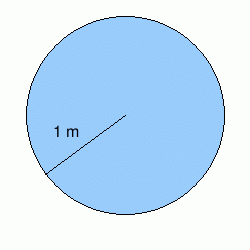Area:

### Complexity=6, Mode=exact

Find the area in terms of π. Figures are not drawn to scale.
Type "pi" in for π. Example: "7π m2" as "7pi sq m".

 1.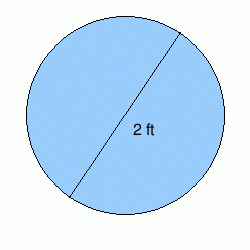Area: 2.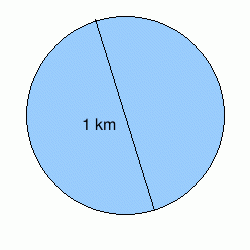Area:

### Complexity=8, Mode=exact

Find the area in terms of π. Figures are not drawn to scale.
Type "pi" in for π. Example: "7π m2" as "7pi sq m".

 1.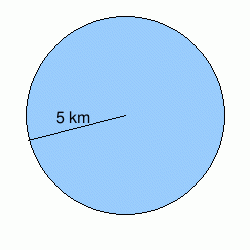Area: 2.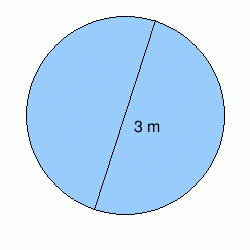Area:

### Complexity=4, Mode=fraction

Find the area. Use 22/7 as an approximation for π, but give the final answer in decimal.
A typed answer would look something like this: 14.5 sq cm.

 1.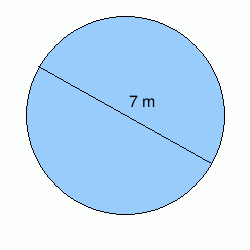Area: 2.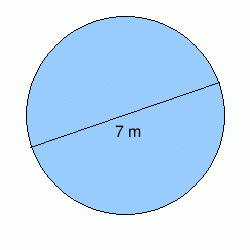Area:

### Complexity=4

Find the area. Use 3.14 as an approximation for π.
A typed answer would look something like this: 14.5 sq cm.

 1.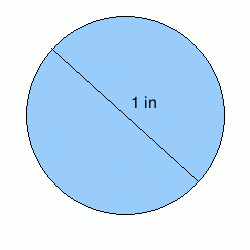Area: 2.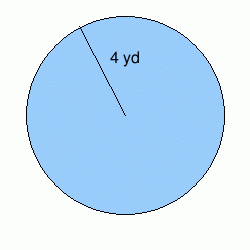Area:

### Complexity=4, Mode=exact

Find the area in terms of π. Figures are not drawn to scale.
Type "pi" in for π. Example: "7π m2" as "7pi sq m".

1Area:
Solution
A = πr2
Since we have that the diameter is 2 yd, we divide it by 2 to get the radius which then is 1 yd.
Plugging values into the equation, we have:
A = π(1 yd))2
A = π(1 yd2)
A = 1π yd2
2Area:
Solution
A = πr2
Plugging values into the equation, we have:
A = π(1 m))2
A = π(1 m2)
A = 1π m2

### Complexity=6, Mode=exact

Find the area in terms of π. Figures are not drawn to scale.
Type "pi" in for π. Example: "7π m2" as "7pi sq m".

1Area:
Solution
A = πr2
Since we have that the diameter is 2 ft, we divide it by 2 to get the radius which then is 1 ft.
Plugging values into the equation, we have:
A = π(1 ft))2
A = π(1 ft2)
A = 1π ft2
2Area:
Solution
A = πr2
Since we have that the diameter is 1 km, we divide it by 2 to get the radius which then is 0.5 km.
Plugging values into the equation, we have:
A = π(1 / 2 km))2
A = π(0.25 km2)
A = 0.25π km2

### Complexity=8, Mode=exact

Find the area in terms of π. Figures are not drawn to scale.
Type "pi" in for π. Example: "7π m2" as "7pi sq m".

1Area:
Solution
A = πr2
Plugging values into the equation, we have:
A = π(5 km))2
A = π(25 km2)
A = 25π km2
2Area:
Solution
A = πr2
Since we have that the diameter is 3 m, we divide it by 2 to get the radius which then is 1.5 m.
Plugging values into the equation, we have:
A = π(3 / 2 m))2
A = π(2.25 m2)
A = 2.25π m2

### Complexity=4, Mode=fraction

Find the area. Use 22/7 as an approximation for π, but give the final answer in decimal.
A typed answer would look something like this: 14.5 sq cm.

1Area:
Solution
A = πr2
Since we have that the diameter is 7 m, we divide it by 2 to get the radius which then is 3.5 m.
Plugging values into the equation, we have:
A = π(7 / 2 m))2
A ≈ (22/7) × (49 / 4 m2)
A ≈ 38.5 m2
2Area:
Solution
A = πr2
Since we have that the diameter is 7 m, we divide it by 2 to get the radius which then is 3.5 m.
Plugging values into the equation, we have:
A = π(7 / 2 m))2
A ≈ (22/7) × (49 / 4 m2)
A ≈ 38.5 m2

### Complexity=4

Find the area. Use 3.14 as an approximation for π.
A typed answer would look something like this: 14.5 sq cm.

1Area:
Solution
A = πr2
Since we have that the diameter is 1 in, we divide it by 2 to get the radius which then is 0.5 in.
Plugging values into the equation, we have:
A = π(1 / 2 in))2
A ≈ 3.14 × (0.25 in2)
A ≈ 0.785 in2
2Area:
Solution